### Home > CALC > Chapter 5 > Lesson 5.5.2 > Problem5-171

5-171.
1. Find the derivative of each of the following functions. Homework Help ✎

1. f(x) = (2x + l)2(3x − l)3

2.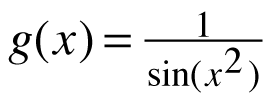3. f(x) = x tan(x2)

4.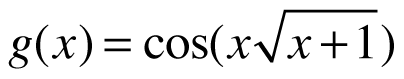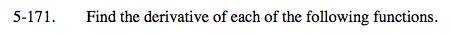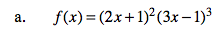Use the Chain Rule combined with the Product Rule.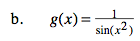Though you could use the Quotient Rule (combined with the Chain Rule), spare yourself the trouble!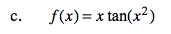f '(x) = tan(x2) + 2x2sec2(x2)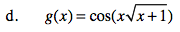Use the Chain Rule combined with the Product Rule.